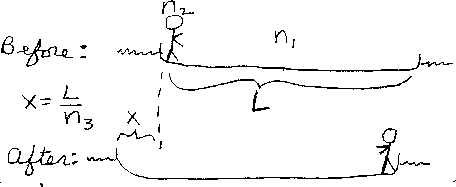Problem C14: A boy of mass n2 is sitting at the extreme left edge of a boat that has a mass of n1. The length of the boat is L. The boy then moves to the extreme right edge of the boat, and the boat moves to the left. How much does the boat move to the left? If the boat moves a distance of x=L/n3, what is the value of n3. Note that n1 and n2 have the same units of mass. n3 is unitless. Assume that there is no horizontal friction between the water and the boat.n1 = n2 = Input n3:
If you are currently in my class, you can record your grade by entering your name and student ID number (without the leading zeros) below and clicking on "record grade".
 First Name = Last Name = ID = Problem: